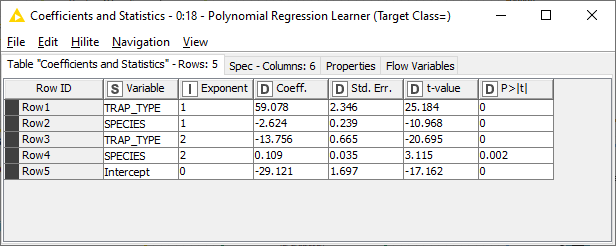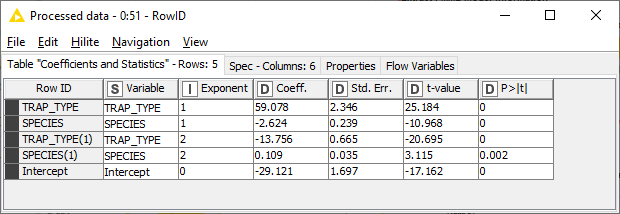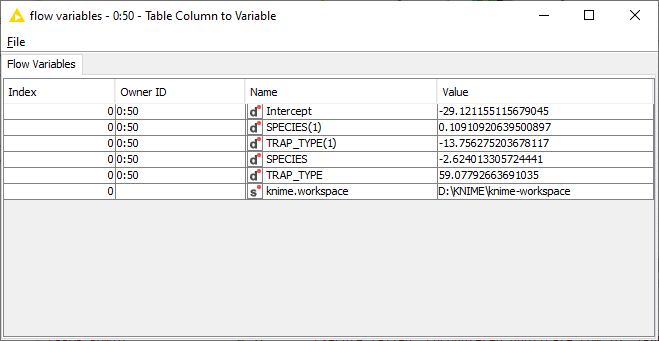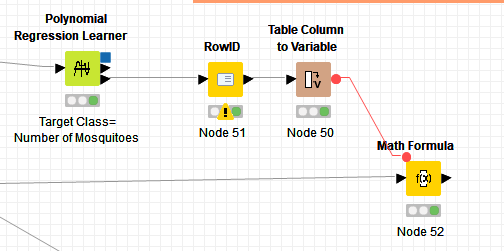# How to convert the Polynomial Regression Learner curves to math formula?

Hi,

After the preparing, cleaning and processing datasets, I want to use polynomial regression leaner and predictor to visualize the curves.The scatter plot visualization is finished, I have no idea how to convert the graph into math formula eg. y=a1+a2x+a3x^2…

Bg

1 Like

The last output of the Polynomial Regression node would give you the coefficients directly.

This is an example from the workflow that @aworker posted:From here one could use a RowID node to identify each row (ensure uniqueness is checked):Then use a Table Column to Variable node to have access to each variable:A Math Node can put it all together and apply the formula to incoming data:

The end result is the same as if you used the Regression Predictor node, so we’re on the right track:Instead of a Math Formula node you could use a String Manipulation node to compile a formula using whatever variable names you’d like.

4 Likes

Thanks @elsamuel for bringing this information. I should have verified this 3rd output port before posting my answer!

And thanks for providing the right answer based on a math formula.

Ael

Hi @elsamuel @aworker Thank you.What is the input data port connected to Math Formula node?
I can not find the flow variable in Math Formula after Table Column to Variable. Is there any reference workflow, the link in first reply was deleted.
Maybe the config of Table Column to Variable node is enough, honestly, I haven’t process the flow variable once yet.Best regard,
Ken

1 Like

Hi @Kenyx

Please find below the workflow with the suggestion by @elsamuel

Best regards

Ael

1 Like

Merci
Ken

1 Like

Avec plaisir @Kenyx !

Kudos should go to @elsamuel who brought the solution!

Best wishes

Ael

This topic was automatically closed 7 days after the last reply. New replies are no longer allowed.